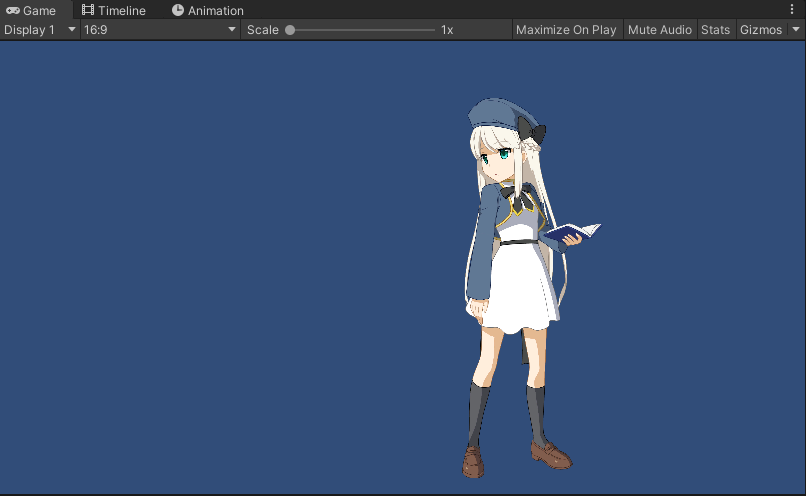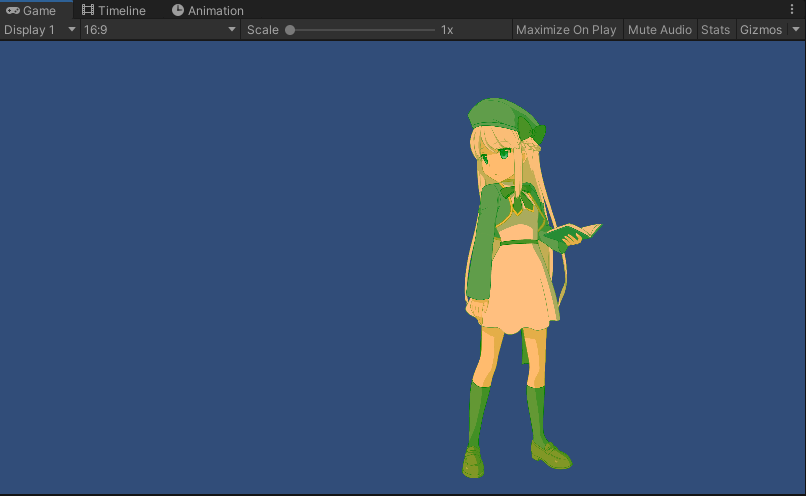# [Unity] Multiply Color/Screen Color

Updated: 05/19/2020

By applying Multiply Color and Screen Color to the model, tints can be changed in real time.
Multiply Color and Screen Color set in the Cubism Editor will be applied without any additional coding by using the Cubism 4.2 or later SDK for Unity.
See the “Multiply Color/Screen Color” page in the Editor manual for details on setting the Multiply Color and Screen Color in the Cubism Editor.

In addition, by coding as needed, it is possible to manipulate Multiply Color and Screen Color from the SDK and perform the following operations.

• Apply Multiply Color and Screen Color interactively.
• Apply Multiply Color and Screen Color settings that have not been set in the Cubism Editor.
• Disable Multiply Color and Screen Color set in the Cubism Editor.

The following is an explanation of the procedure.

## Procedures

The process is as follows.

• Model placement
• Overwrite flag settings for Multiply Color and Screen Color
• Multiply Color and Screen Color settings

### Model placement

Place the model for which you want to set the Multiply Color and Screen Color in any scene.

### Overwrite flag settings for Multiply Color and Screen Color

Set the overwrite flag for Multiply Color and Screen Color to true.
The default is false, which means that color information from the model is used.

There are two types of overwrite flags: one for the entire model, which is held by [CubismRenderController], and one for each Drawable object, which is held by [CubismRenderer].

Sample code is shown below.
It is assumed that the script is attached to the root object of the model as a component.

```// Get CubismRenderController Component
CubismRenderController renderController =　GetComponent<CubismRenderController>();

// Manipulate flags across the entire model.
renderController.OverwriteFlagForModelMultiplyColors = true;
renderController.OverwriteFlagForModelScreenColors = true;

// Manipulation of individual Drawable flags.
renderController.Renderers.OverwriteFlagForMultiplyColors = true;
renderController.Renderers.OverwriteFlagForScreenColors = true;```

If the Overwrite flag for the entire model is enabled, it is possible to manipulate the Multiply Color and Screen Color from the SDK even if the Overwrite flag for an individual Drawable is disabled.

[CubismRenderController] is added as a component to the model root object and can be obtained by using GetComponent.
[CubismRenderController] allocates an array of [CubismRenderer] for each Drawable object in the model, and each [CubismRenderer] can be referenced from [CubismRenderController].

### Multiply Color and Screen Color settings

Define Multiply Color and Screen Color and set them to the model.
The code below shows the set values for setting red for the Multiply Color and green for the Screen Color for all Drawables.
The set color is stored in each [CubismRenderer] as a UnityEngine.Color type.
In the example below, it is set in RGBA, but A is not used in the calculation of Multiply Color and Screen Color.

```var multiplyColor = new Color(1.0f, 0.5f, 0.5f, 1.0f);
var screenColor = new Color(0.0f, 0.5f, 0.0f, 1.0f);

for (var i = 0; i < RenderController.Renderers.Length; i++)
{
// MultiplyColor
renderController.Renderers[i].MultiplyColor = multiplyColor;
// ScreenColor
renderController.Renderers[i].ScreenColor = screenColor;
}```

Before applying Multiply Color and Screen ColorAfter applying red to Multiply Color and green to Screen ColorThe model’s Multiply Color and Screen Color process is updated in all Drawables when there is a change in color information.

###### Tips

In this case, the same Multiply Color and Screen Color are set for all Drawables, but it is possible to set different Multiply Color and Screen Color for each Drawable.
To disable Multiply Color and Screen Color, set the following.

Multiply Color (1.0, 1.0, 1.0, 1.0)
Screen Color (0.0, 0.0, 0.0, 1.0)

## Other Related Functions and Processes

If a model parameter is associated with a change in Multiply Color/Screen Color, the model may change the Multiply Color/Screen Color when the model is animated, rather than from the SDK side.

The property IsBlendColorDirty is implemented in [CubismDynamicDrawableData] to indicate that the Multiply Color and/or Screen Color have been changed.

This property is a flag that is set when either the Multiply Color or Screen Color is changed on the model side, and does not determine whether the Multiply Color or Screen Color is changed.

To handle [CubismDynamicDrawableData] data, the event OnDynamicDrawableData of [CubismModel] must be used.

Registering functions

```// Model
CubismModel model;

// Register listener.
model.OnDynamicDrawableData += OnDynamicDrawableData;```

Example of function to register

```private void OnDynamicDrawableData(CubismModel sender, CubismDynamicDrawableData[] data)
{
for (int i = 0; i < data.Length; i++)
{
if (data[i].IsBlendColorDirty)
{
Debug.Log(i + ": Did Changed.");
}
}
}```

### Apply Multiply Color and Screen Color to shaders

Some additional implementation is required when using Multiply Color/Screen Color in customized shaders.
When using [CubismRenderer] and [CubismRenderController], it is easily implemented by adding the following properties and processing to the shader.

```[PerRendererData] cubism_MultiplyColor("Multiply Color", Color) = (1.0, 1.0, 1.0, 1.0)
[PerRendererData] cubism_ScreenColor("Screen Color", Color) = (0.0, 0.0, 0.0, 1.0)```
```sampler2D _MainTex;
fixed4 cubism_MultiplyColor;
fixed4 cubism_ScreenColor;

```fixed4 frag (v2f IN) : SV_Target
{
fixed4 textureColor = tex2D(_MainTex, IN.texcoord);

// Multiply
textureColor.rgb *= cubism_MultiplyColor.rgb;

// Screen
textureColor.rgb = (textureColor.rgb + cubism_ScreenColor.rgb) - (textureColor.rgb * cubism_ScreenColor.rgb);

fixed4 OUT = textureColor * IN.color;

// Apply Cubism alpha to color.
CUBISM_APPLY_ALPHA(IN, OUT);

return OUT;
}```